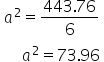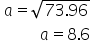Maths-
General
Easy

Question

# Surface area of a cube is 443.76 cm2 . Find the volume of it?

## 7.68.48.66.4Hint:

## The correct answer is: 6.4

### We are given that  Surface area of a cube = 443.76 cm2 We have to find volume of the cube 6a2 = 443.76 Divide both sides of equation by 6Taking square root of both sides we get,Therefore, the volume of the given cube is Volume = a3 = (8.6)3Volume = 636.056 cm3 The approximate of the volume is 6.4 from the given options, Therefore the correct option is d) 6.4.#### With Turito Foundation.#### Get an Expert Advice From Turito.